Get instant live expert help with Excel or Google Sheets“My Excelchat expert helped me in less than 20 minutes, saving me what would have been 5 hours of work!”

#### Post your problem and you’ll get Expert help in seconds.

Your message must be at least 40 characters
Our professional Expert are available now. Your privacy is guaranteed.

# The Excel N Function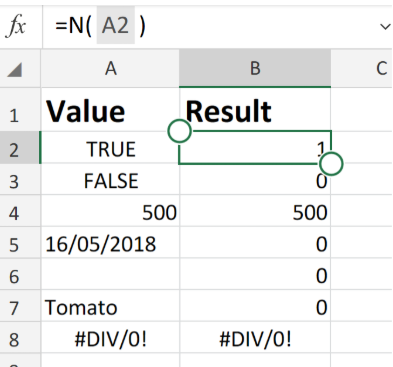Figure 1. of Excel N Function

The purpose of the Excel N Function is to convert a value to a number. This is an in-built Function in Excel which we can categorize as an Information Function.

## Operation Syntax

N(value)

The parameters of our formula/syntax are as follows

• Value – A data value which we wish to convert to a number.

## Note the following conditions

1. If our data value = a number, our N Function will be determined as a number value.
2. If our data value = a calendar date, our N Function will be determined as a serial number.
3. If our data value = True, our N Function will be determined as 1.
4. If our data value = False, our N Function will be determined as 0.
5. If our data value = Error, our N Function will be determined as an Error.
6. For any and all other types of data value, our N Function will be determined as 0!, or same.

## How to use the N Function in Excel

Based on the Excel worksheets displayed below, the attached N operation syntax would be determined as;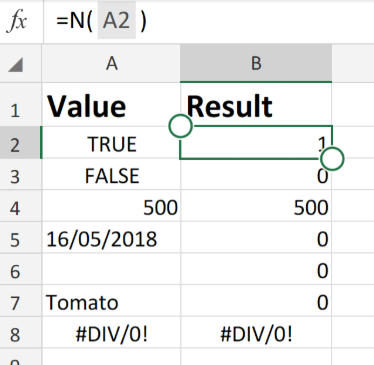Figure 2. of Excel N Function

`=N(A2)`

-Determined as 1.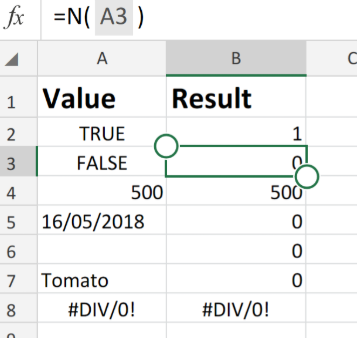Figure 3. of Excel N Function.

`=N(A3)`

-Determined as 0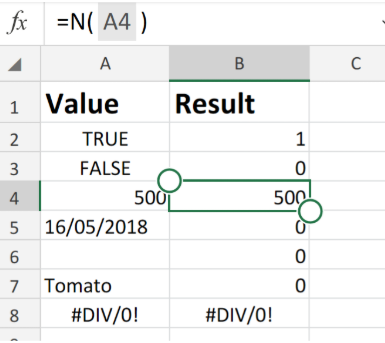Figure 4. of Excel N Function.

`=N(A4)`

-Determined as 500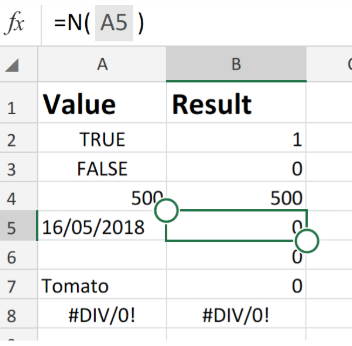Figure 5. of Excel N Function.

`=N(A5)`

-Determined as 0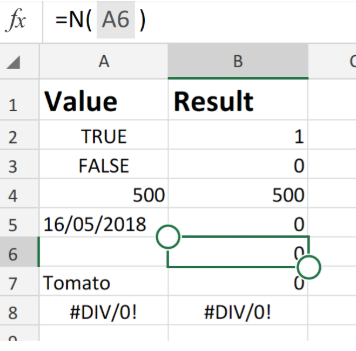Figure 6. of Excel N Function.

`=N(A6)`

-Determined as 0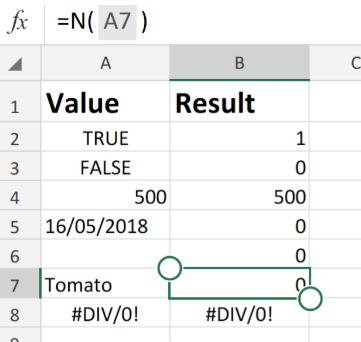Figure 7. of Excel N Function.

`=N(A7)`

-Determined as 0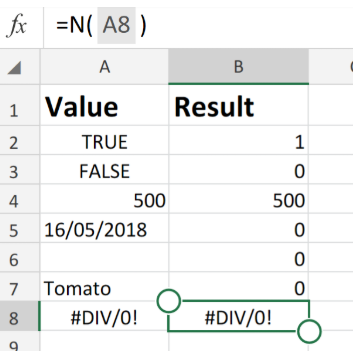Figure 8. of Excel N Function.

`=N(A8)`

-Determined as same value/0!

The Excel N Function is a worksheet Function and is usually entered into an Excel worksheet as part of a formula.

## Instant Connection to an Expert through our Excelchat Service

Our live Excelchat Service is here for you. We have Excel Experts available 24/7 to answer any Excel questions you may have. Guaranteed connection within 30 seconds and a customized solution for you within 20 minutes

### Did this post not answer your question? Get a solution from connecting with the expert.Another blog reader asked this question today on Excelchat:## Subscribe to Excelchat.coAnother blog reader asked this question today on Excelchat: